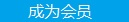您可以捐助，支持我们的公益事业。 1元 10元 50元 认证码：必填求知 文章 文库 Lib 视频 iProcess 课程 认证 咨询 工具 讲座 Modeler Code要资料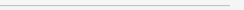订阅捐助
OpenGL核心技术之GPU编程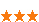966 次浏览     评价： 好 中 差
2018-8-31

 #version 330 core layout (location = 0) in vec3 position; layout (location = 1) in vec2 texCoords; uniform mat4 model; uniform mat4 view; uniform mat4 projection; out VS_OUT { vec2 TexCoords; } vs_out; void main() { gl_Position = projection * view * model * vec4(position, 1.0f); vs_out.TexCoords = texCoords; }

 #version 330 core out vec4 color; in VS_OUT { vec2 TexCoords; } fs_in; uniform sampler2D texture; void main() { color = texture(texture, fs_in.TexCoords); }

OpenGL为我们提供了一个叫做uniform缓冲对象(Uniform Buffer Object)的工具，使我们能够声明一系列的全局uniform变量， 它们会在几个着色器程序中保持一致。当时用uniform缓冲的对象时相关的uniform只能设置一次。我们仍需为每个着色器手工设置唯一的uniform。创建和配置一个uniform缓冲对象需要费点功夫。

 #version 330 core layout (location = 0) in vec3 position; layout (std140) uniform Matrices { mat4 projection; mat4 view; }; uniform mat4 model; void main() { gl_Position = projection * view * model * vec4(position, 1.0); }

 layout (std140) uniform ExampleBlock { float value; vec3 vector; mat4 matrix; float values; bool boolean; int integer; };

GLSL 默认使用的uniform内存布局叫做共享布局(shared layout)，叫共享是因为一旦偏移量被硬件定义，它们就会持续地被多个程序所共享。使用共享布局，GLSL可以为了优化而重新放置uniform变量，只要变量的顺序保持完整。因为我们不知道每个uniform变量的偏移量是多少，所以我们也就不知道如何精确地填充uniform缓冲。我们可以使用像glGetUniformIndices这样的函数来查询这个信息，但是这超出了本节教程的范围。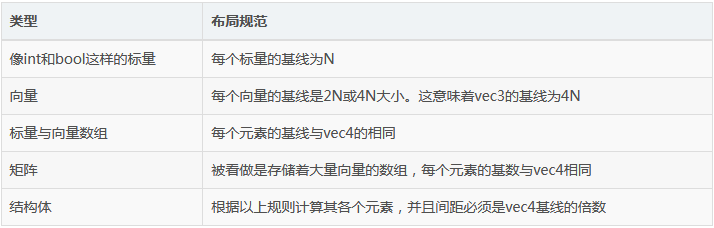layout (std140) uniform ExampleBlock { // base alignment ---------- // aligned offset float value; // 4 // 0 vec3 vector; // 16 // 16 (必须是16的倍数，因此 4->16) mat4 matrix; // 16 // 32 (第 0 行) // 16 // 48 (第 1 行) // 16 // 64 (第 2 行) // 16 // 80 (第 3 行) float values; // 16 (数组中的标量与vec4相同)//96 (values) // 16 // 112 (values) // 16 // 128 (values) bool boolean; // 4 // 144 int integer; // 4 // 148 };

 GLuint uboExampleBlock; glGenBuffers (1, &uboExampleBlock); glBindBuffer (GL_UNIFORM_BUFFER, uboExampleBlock); glBufferData (GL_UNIFORM_BUFFER, 150, NULL, GL_ STATIC_ DRAW); // 分配150个字节的内存空间 glBindBuffer (GL_UNIFORM_BUFFER, 0);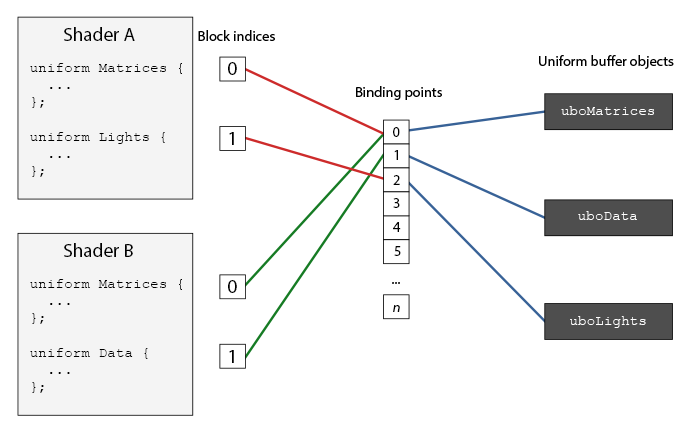GLuint lights_index = glGetUniformBlockIndex (shaderA . Program , "Lights"); glUniformBlockBinding (shaderA.Program, lights_index, 2 );

 layout(std140, binding = 2) uniform Lights { ... };

 glBindBufferBase(GL_UNIFORM_BUFFER, 2, uboExampleBlock ); // 或者 glBindBufferRange (GL_UNIFORM_BUFFER, 2, uboExample Block , 0, 150);

 glBindBuffer (GL_ UNIFORM_BUFFER, uboExampleBlock); GLint b = true; // GLSL中的布尔值是4个字节，因此我们将它创建为一个4字节的整数 glBufferSubData (GL_ UNIFORM_BUFFER, 142, 4, &b); glBindBuffer (GL_UNIFORM_BUFFER, 0);

 #version 330 core layout (location = 0) in vec3 position; layout (std140) uniform Matrices { mat4 projection; mat4 view; }; uniform mat4 model; void main() { gl_Position = projection * view * model * vec4(position, 1.0); }

 GLuint uniformBlockIndexRed = glGetUniformBlockIndex (shaderRed .Program, "Matrices"); GLuint uniformBlockIndexGreen = glGetUniformBlockIndex (shaderGreen .Program, "Matrices"); GLuint uniformBlockIndexBlue = glGetUniformBlockIndex (shaderBlue .Program, "Matrices"); GLuint uniformBlockIndexYellow = glGetUniformBlock Index (shaderYellow.Program, "Matrices"); glUniform BlockBinding (shaderRed.Program, uniformBlock IndexRed, 0); glUniform BlockBinding (shaderGreen.Program, uniformBlockIndexGreen, 0); glUniformBlockBinding (shaderBlue.Program, uniformBlockIndexBlue, 0); glUniformBlockBinding (shaderYellow.Program, uniformBlockIndexYellow, 0);

 GLuint uboMatrices glGenBuffers( 1, &uboMatrices); glBindBuffer (GL_UNIFORM_BUFFER, uboMatrices); glBufferData( GL_UNIFORM_BUFFER, 2 * sizeof(glm: :mat4 ) , NULL, GL_STATIC_DRAW); glBindBuffer (GL_UNIFORM_BUFFER, 0); glBindBufferRange (GL_ UNIFORM_ BUFFER, 0, uboMatrices , 0, 2 * sizeof (glm::mat4));

 glm::mat4 projection = glm::perspective(45.0f, (float )width / (float)height, 0.1f, 100.0f); glBindBuffer (GL_UNIFORM_BUFFER, uboMatrices); glBufferSubData (GL_UNIFORM_BUFFER, 0, sizeof (glm: : mat4 ) , glm::value_ptr(projection)); glBindBuffer (GL_UNIFORM_BUFFER, 0);

 glm::mat4 view = camera.GetViewMatrix(); glBindBuffer (GL_UNIFORM_BUFFER, uboMatrices); glBufferSubData( GL_ UNIFORM_ BUFFER, sizeof(glm::mat4), sizeof (glm: : mat4) , glm:: value_ptr(view)); glBindBuffer (GL_UNIFORM_BUFFER, 0);

 glBindVertexArray(cubeVAO); shaderRed.Use(); glm::mat4 model; model = glm:: translate(model, glm::vec3(-0.75f, 0.75f , 0.0f )); // 移动到左上方 glUniformMatrix4fv(modelLoc, 1, GL_FALSE, glm:: value_ ptr (model)); glDrawArrays (GL_TRIANGLES, 0, 36); // ... 绘制绿色立方体 // ... 绘制蓝色立方体 // ... 绘制黄色立方体 glBindVertexArray(0);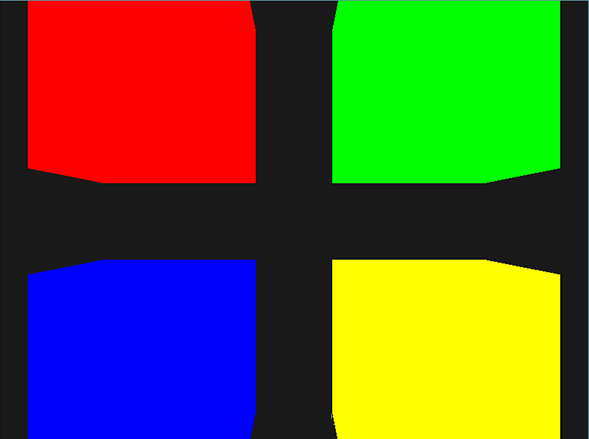// Clear buffers glClearColor (0.1f, 0.1f, 0.1f, 1.0f); glClear (GL_COLOR_ BUFFER_BIT | GL_DEPTH_BUFFER_BIT); // Set the view and projection matrix in the uniform block - we only have to do this once per loop iteration . glm::mat4 view = camera.GetViewMatrix(); glBindBuffer (GL_ UNIFORM_BUFFER, uboMatrices); glBufferSubData (GL_UNIFORM_ BUFFER, sizeof (glm::mat4 ) , sizeof (glm::mat4), glm:: value_ptr(view)); glBindBuffer (GL_UNIFORM_BUFFER, 0); // Draw 4 cubes // RED glBindVertexArray(cubeVAO); shaderRed.Use(); glm::mat4 model; model = glm::translate (model, glm::vec3 (-0.75f, 0.75f , 0.0f)); // Move top-left glUniformMatrix4fv (glGetUniformLocation (shaderRed . Program, " model "), 1, GL_ FALSE, glm ::value_ ptr (model )); glDrawArrays (GL_ TRIANGLES, 0, 36); // GREEN shaderGreen.Use(); model = glm::mat4(); model = glm::translate (model, glm::vec3(0.75f, 0.75f, 0.0f )); // Move top-right glUniformMatrix4fv (glGetUniformLocation (shaderGreen. Program, "model"), 1, GL_ FALSE, glm::value_ptr (model )); glDrawArrays (GL_ TRIANGLES, 0, 36); // BLUE shaderBlue.Use(); model = glm::mat4(); model = glm::translate (model, glm::vec3 (-0.75f, -0.75f , 0.0f )); // Move bottom-left glUniformMatrix4fv (glGetUniformLocation (shaderBlue .Program , "model "), 1, GL_FALSE, glm::value_ ptr (model )); glDrawArrays (GL_TRIANGLES, 0, 36); // YELLOW shaderYellow.Use(); model = glm::mat4(); model = glm::translate (model, glm::vec3 (0.75f, - 0.75f , 0.0f )); // Move bottom-right glUniformMatrix4fv (glGetUniformLocation (shaderYellow .Program , "model "), 1, GL_ FALSE, glm ::value_ptr ( model )); glDrawArrays (GL_ TRIANGLES, 0, 36); glBindVertexArray(0);966 次浏览  评价: 好 中 差订阅捐助
 相关文章 云计算的架构 对云计算服务模型 云计算核心技术剖析 了解云计算的漏洞
 相关文档 云计算简介 云计算简介与云安全 下一代网络计算--云计算 软浅析云计算
 相关课程 云计算原理与应用 云计算应用与开发 CMMI体系与实践 基于CMMI标准的软件质量保证每天2个文档/视频 扫描微信二维码订阅
 订阅技术月刊 获得每月300个技术资源